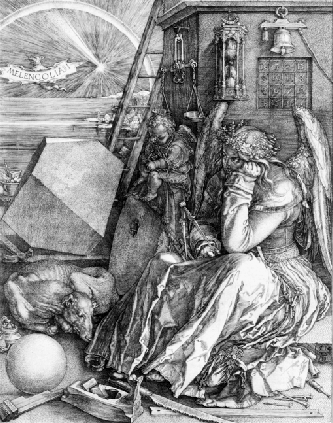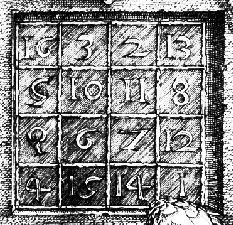# Matrices and Magic Squares in Matlab

Posted by SUYOG PATIL on 7:27 PM in , , ,This image is filled with mathematical symbolism, and if you look carefully,
you will see a matrix in the upper right corner. This matrix is known as a
magic square and was believed by many in Dürer’s time to have genuinely
magical properties. It does turn out to have some fascinating characteristics
worth exploring.Entering Matrices
The best way for you to get started with MATLAB is to learn how to handle
matrices. Start MATLAB and follow along with each example.
You can enter matrices into MATLAB in several different ways:
• Enter an explicit list of elements.
• Load matrices from external data files.
• Generate matrices using built-in functions.
• Create matrices with your own functions and save them in files.
Start by entering Dürer’s matrix as a list of its elements. You only have to
• Separate the elements of a row with blanks or commas.
• Use a semicolon, ; , to indicate the end of each row.
• Surround the entire list of elements with square brackets, [ ].
To enter Dürer’s matrix, simply type in the Command Window
A = [16 3 2 13; 5 10 11 8; 9 6 7 12; 4 15 14 1]
MATLAB displays the matrix you just entered:
A =
16 3 2 13
5 10 11 8
9 6 7 12
4 15 14 1
This matrix matches the numbers in the engraving. Once you have entered
the matrix, it is automatically remembered in the MATLAB workspace. You
can refer to it simply as A. Now that you have A in the workspace, take a look
at what makes it so interesting. Why is it magic?
sum, transpose, and diag
You are probably already aware that the special properties of a magic square
have to do with the various ways of summing its elements. If you take the
sum along any row or column, or along either of the two main diagonals,
you will always get the same number. Let us verify that using MATLAB.
The first statement to try is
sum(A)
MATLAB replies with
ans =
34 34 34 34
When you do not specify an output variable, MATLAB uses the variable ans,
short for answer, to store the results of a calculation. You have computed a
row vector containing the sums of the columns of A. Each of the columns has
the same sum, the magic sum, 34.
How about the row sums? MATLAB has a preference for working with the
columns of a matrix, so one way to get the row sums is to transpose the
matrix, compute the column sums of the transpose, and then transpose the
result. For an additional way that avoids the double transpose use the
dimension argument for the sum function.
MATLAB has two transpose operators. The apostrophe operator (e.g., A')
performs a complex conjugate transposition. It flips a matrix about its main
diagonal, and also changes the sign of the imaginary component of any
complex elements of the matrix. The dot-apostrophe operator (e.g., A.'),
transposes without affecting the sign of complex elements. For matrices
containing all real elements, the two operators return the same result.
So
A'
produces
ans =
16 5 9 4
3 10 6 15
2 11 7 14
13 8 12 1
and
sum(A')'
produces a column vector containing the row sums
ans =
34
34
34
34
The sum of the elements on the main diagonal is obtained with the sum and
the diag functions:
diag(A)
produces
ans =
16
10
7
1
and
sum(diag(A))
produces
ans =
34
The other diagonal, the so-called antidiagonal, is not so important
But a function originally intended for use in graphics, fliplr, flips a matrix
from left to right:
sum(diag(fliplr(A)))
ans =
34
You have verified that the matrix in Dürer’s engraving is indeed a magic
square and, in the process, have sampled a few MATLAB matrix operations.
The following sections continue to use this matrix to illustrate additional
MATLAB capabilities.

Subscripts
The element in row i and column j of A is denoted by A(i,j). For example,
A(4,2) is the number in the fourth row and second column. For the magic
square, A(4,2) is 15. So to compute the sum of the elements in the fourth
column of A, type
A(1,4) + A(2,4) + A(3,4) + A(4,4)
This subscript produces
ans =
34
but is not the most elegant way of summing a single column.
It is also possible to refer to the elements of a matrix with a single subscript,
A(k). A single subscript is the usual way of referencing row and column
vectors. However, it can also apply to a fully two-dimensional matrix, in
which case the array is regarded as one long column vector formed from the
columns of the original matrix. So, for the magic square, A(8) is another way
of referring to the value 15 stored in A(4,2).
If you try to use the value of an element outside of the matrix, it is an error:
t = A(4,5)
Index exceeds matrix dimensions.
Conversely, if you store a value in an element outside of the matrix, the size
increases to accommodate the newcomer:
X = A;
X(4,5) = 17
X =
16 3 2 13 0
5 10 11 8 0
9 6 7 12 0
4 15 14 1 17
The Colon Operator
The colon, :, is one of the most important MATLAB operators. It occurs in
several different forms. The expression
1:10
is a row vector containing the integers from 1 to 10:
1 2 3 4 5 6 7 8 9 10
To obtain nonunit spacing, specify an increment. For example,
100:-7:50
is
100 93 86 79 72 65 58 51
and
0:pi/4:pi
is
0 0.7854 1.5708 2.3562 3.1416
Subscript expressions involving colons refer to portions of a matrix:
A(1:k,j)
is the first k elements of the jth column of A. Thus:
sum(A(1:4,4))
computes the sum of the fourth column. However, there is a better way to
perform this computation. The colon by itself refers to all the elements in
a row or column of a matrix and the keyword end refers to the last row or
column. Thus:
sum(A(:,end))
computes the sum of the elements in the last column of A:
ans =
34
Why is the magic sum for a 4-by-4 square equal to 34? If the integers from 1
to 16 are sorted into four groups with equal sums, that sum must be
sum(1:16)/4
which, of course, is
ans =
34
The magic Function
MATLAB actually has a built-in function that creates magic squares of almost
any size. Not surprisingly, this function is named magic:
B = magic(4)
B =
16 2 3 13
5 11 10 8
9 7 6 12
4 14 15 1
This matrix is almost the same as the one in the Dürer engraving and has
all the same “magic” properties; the only difference is that the two middle
columns are exchanged.
To make this B into Dürer’s A, swap the two middle columns:
A = B(:,[1 3 2 4])
This subscript indicates that—for each of the rows of matrix B—reorder the
elements in the order 1, 3, 2, 4. It produces:
A =
16  3   2  13
5   10 11  8
9   6   7   12
4  15  14  1